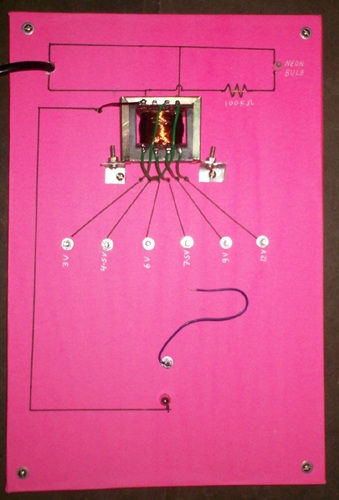top of page

# TransformerPicture of Transformer Model

This is a simple Project for CBSE +2 Students to show how no of turns effects the e.m.f

No. of turns in Primary winding  is _______.

No. of Turns in Secondary winding at each tapping  is _______.

Input Voltage is _________.

Output Voltage in each tapping from common is _________.

In our experiment we have made a Step Down Transformer. In a step down transformer Primary turns is greater than secondary turns. Primary winding of a transformer is always connected to a supply AC source.

For taking input/output voltage  reading of transformer, you can use a voltmeter or Multimeter. For best result use a Digital Multimeter (cost you Rs 120).

For simplicity we have used a small size  12V 2W bulb at output which is commonly used in bike. It glows at every tapping. The brightness increases from lower to higher turns. In my experiment output is from 3V to 12V.

Result: the turns ratio determines the ratio of the voltages in the two coils.

As the volts per turn, which is proportional to the ﬂux in the machine, is the same for the whole winding,

V1 : V2 = T1 : T2

or

V1 / T1 = V2 / T2

You may also be interested in Rectifier Circuit which is commonly used with transformer and converts AC in to DC.

bottom of page